Data Science
Resource

# Learn Introductory Statistics with R

R programming is great for statistical computing and graphics, with powerful packages optimized for data analysis and data visualization.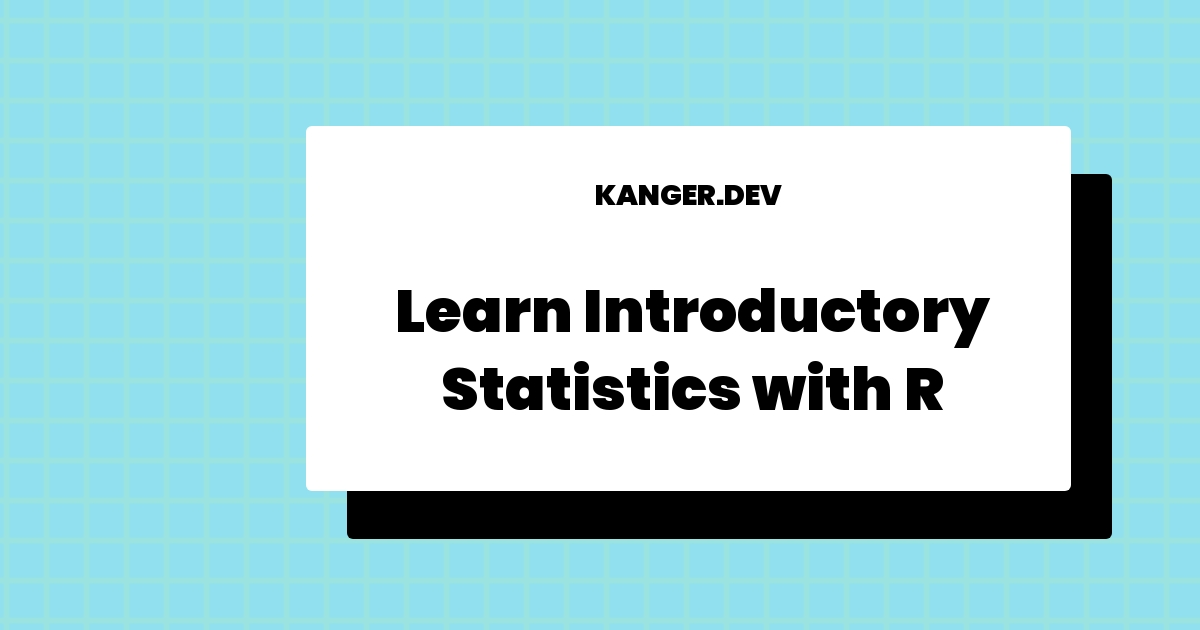Written by
Editors
Updated on
May 10, 2023

R programming is great for statistical computing and graphics, with powerful packages optimized for data analysis and data visualization.

R was originally designed for Statistical analysis, and its clean syntax has led it to be adopted by beginners to create complex statistical models with just a few lines of code.

While there are better options than R for working with things like neural networks and advanced deep learning models, R’s ecosystem is remarkably efficient for Data Science.

R programming is more dedicated to Data Science and statistical computing compared to Python and Julia.

It also offers a range of packages, algorithms, and resources that are useful for complex machine learning tasks, such as time series analysis, classification, clustering, linear modeling, etc.

Most Statisticians and Data Scientists use and contribute to R packages, and you're likely to find support quickly from its diverse community members for any kind of statistical analysis you're struggling to perform.

In this article, we want to suggest some high-quality resources that provide a solid foundation in Essential Statistics Concepts, all while working with data in R programming to clean, analyze, and graph your data.

If you prefer learning from the books, we recommend three books for the beginners.

Most online tutorials on basic statistics rarely cover the essential topics required for data science. If you have a basic familiarity with R programming, these classes will help you learn the most important modeling and prediction techniques, along with important applications for both data science and machine learning.

Let's inspect these courses.

## High-quality Courses to Learn Introductory Statistics with R

These courses will help you learn how to use R with statistical and probabilistic approaches to understand and gain insights from data.

Let's dive in...

### Introduction to Statistics in R

This beginner-level course offered by DataCamp is suitable to learn probability and to conduct a well-designed study to draw your own conclusions from data using R Programming.

Through the guided lectures, you’ll work with the random numbers for experimental probability.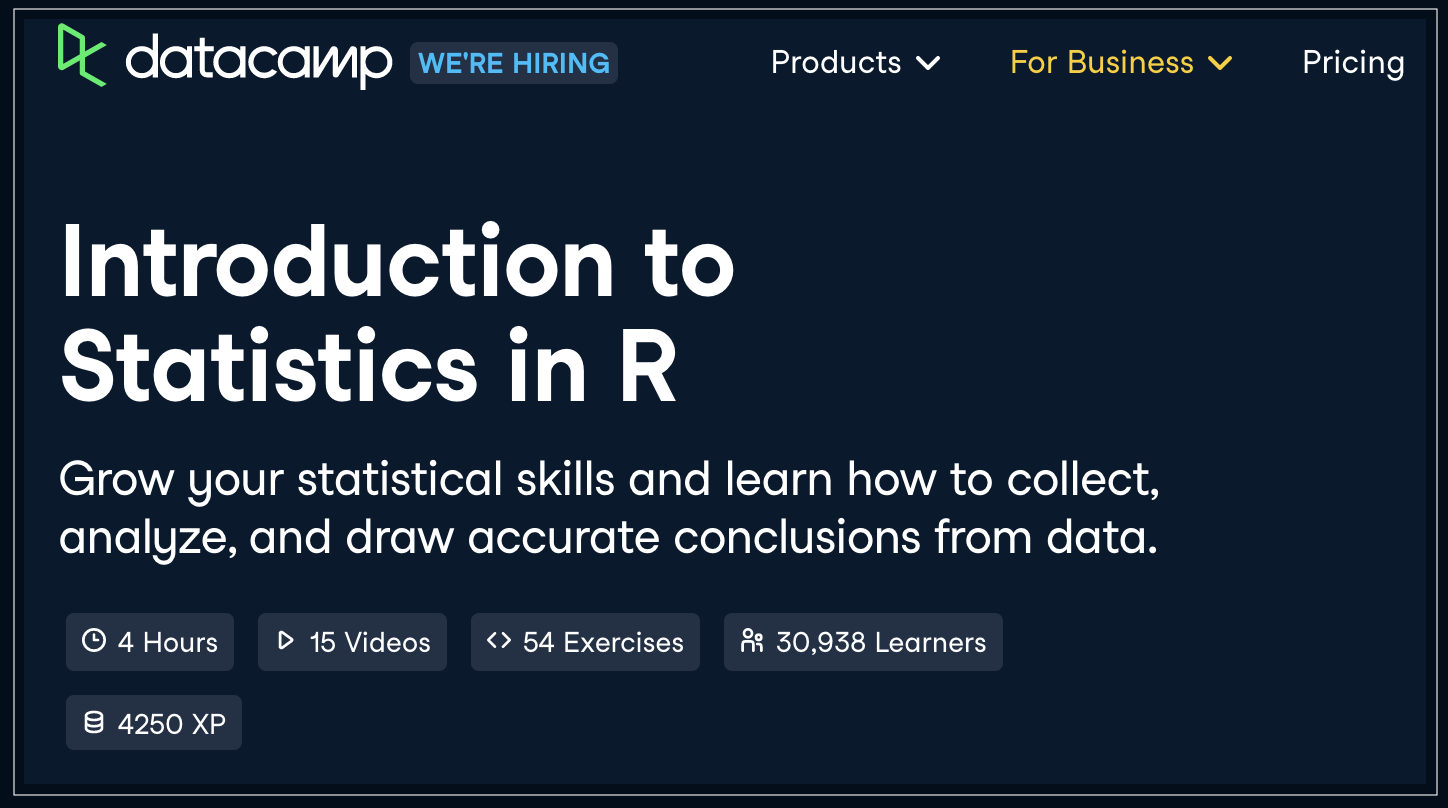You will also grow your understanding of probability distributions and finally, learn correlation and experimental design.

Introduction to Statistics in R

### Conditional Probability in R

This beginner level course is excellent for learning conditional probability, Bayes' theorem, and the Naive Bayes algorithm in R programming.

You will learn to assign probabilities based on conditions, prior knowledge, event independence and create robust spam filters using multinomial Naive Bayes.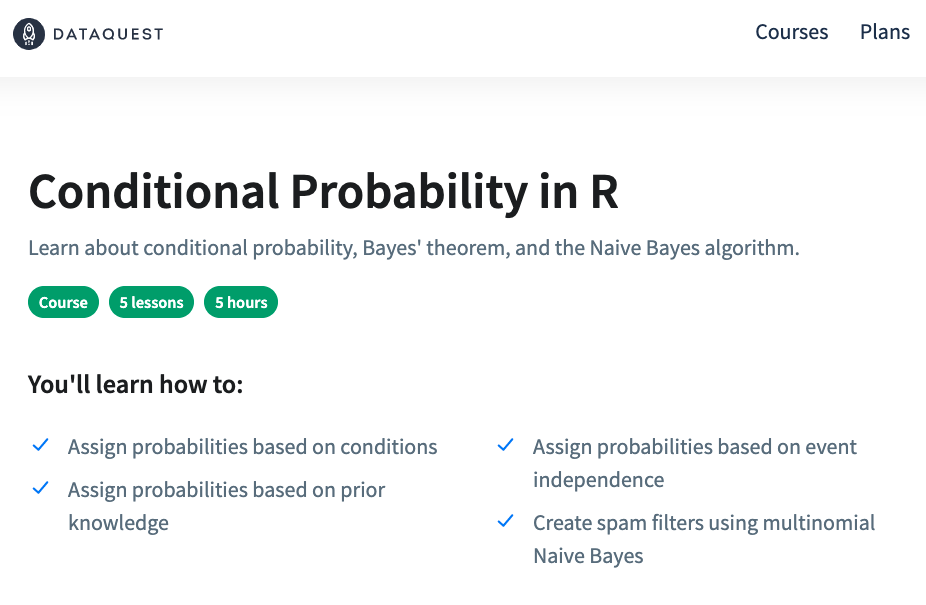In this course, you will learn new techniques to better estimate probabilities in R.

By the end, you will have become equipped to use Statistics for Data Science, including algorithms, to solve real-world problems.

Conditional Probability in R

### Basic Statistics

This beginner-friendly course offered by The University of Amsterdam is suitable for absolute beginners to gain familiarity with the basic statistical concepts for data analysis.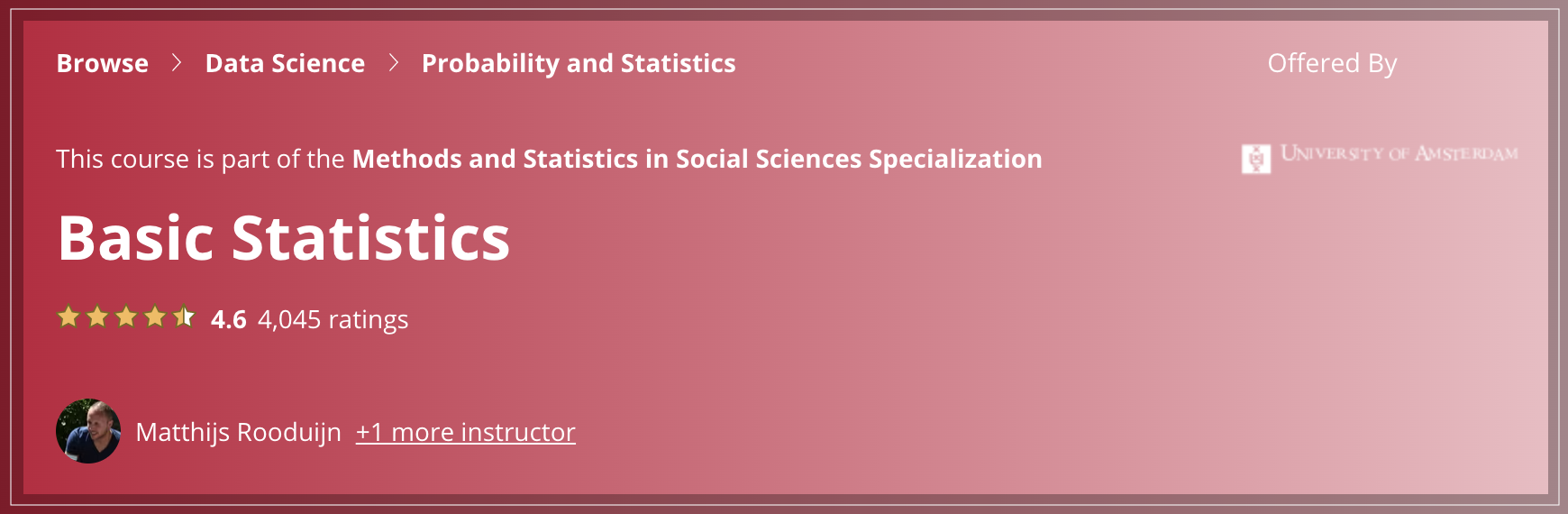You should have a basic knowledge of working with R programming for enrolling in this course.

Upon successful completion, you’ll have gained data science skills in Statistics, Confidence Interval, Statistical Hypothesis Testing, and the basics of R Programming.

Basic Statistics

### Statistics and R

Harvard offers this open course to help learners understand the basic Statistical Concepts with R Programming.

Through the guided lectures, you will gain the R programming skills necessary for analyzing real-world data.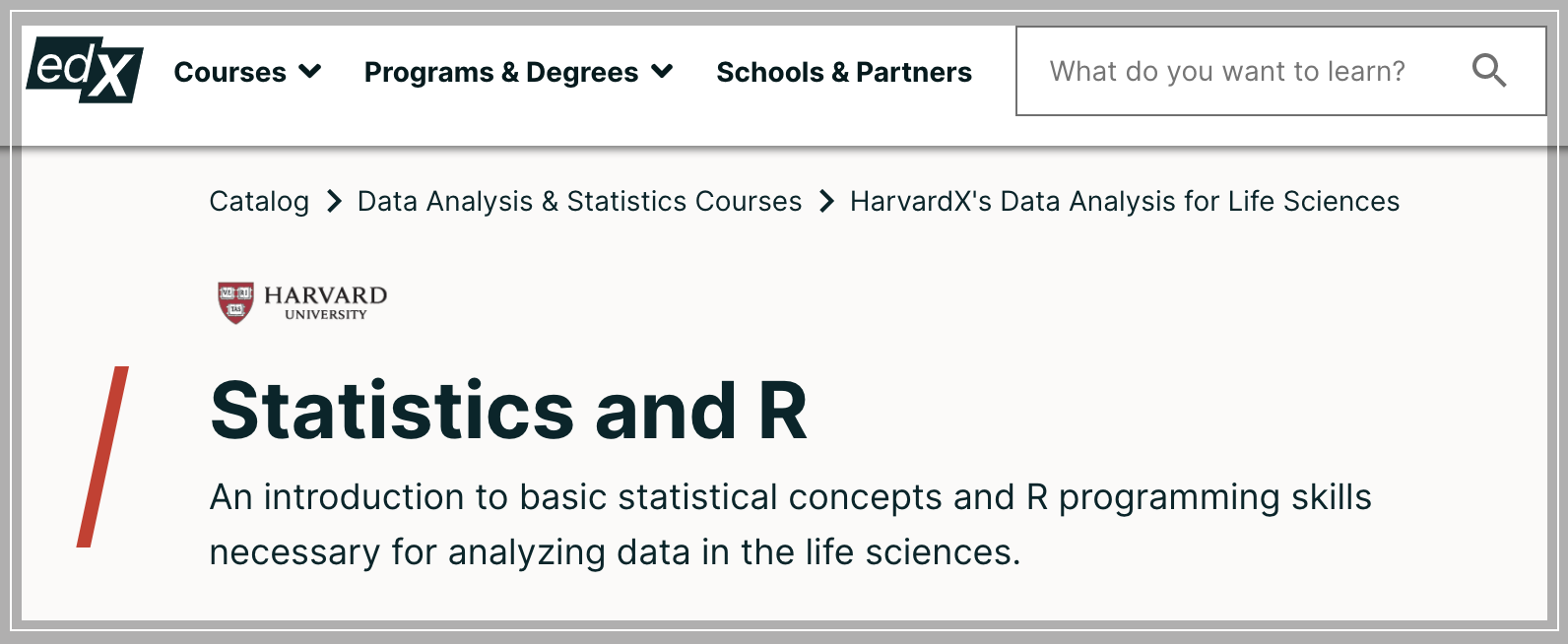By the end of this course, you’ll have gained some foundational knowledge of statistics with R Programming for Data Science.

Statistics and R

### Data Analysis with R Specialization

This beginner-friendly specialization offered by Duke University is suitable for beginners to master statistics with R.

You will learn Exploratory Data Analysis, Statistical Hypothesis Testing, Regression Analysis, Bayesian Linear Regression, Bayesian Inference, and Model Selection.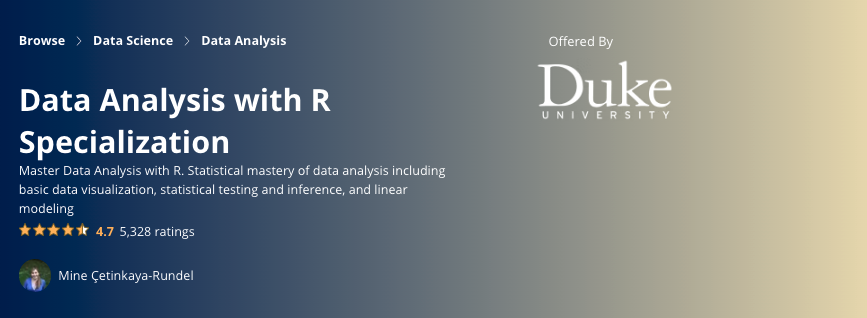Upon successful completion, you will have gained skills in Bayesian Statistics, Linear Regression, Statistical Inference, R Programming, and RStudio.

Statistics with R Specialization

### Statistics with R

This beginner-level course offered on Udemy is suitable for learners who have basic knowledge of R Programming and Statistics.

You’ll also gain skills to Create Histograms and Cumulative Frequency Charts, Perform Pnivariate Analyses, determine skewness and kurtosis, and more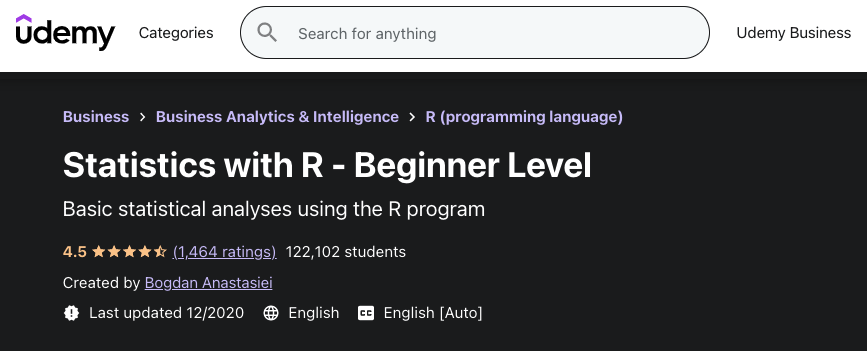By the end of this course, you should be able to manipulate data in R, Compute Statistical Indicators, Build frequency tables.

Statistics with R

### Bayesian Statistics: From Concept to Data Analysis

This course is suitable to help learners advance in Statistical understanding and data analysis skills through the guided lectures and hands-on exercises in R Programming and Excel.

Through the series of lectures, demonstrations, readings, exercises, and discussions, you’ll know how to perform data analysis on your own.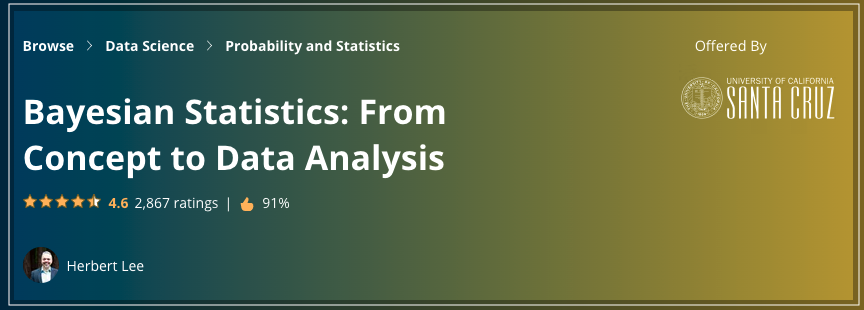By the end, you’ll have a sound understanding of the Bayesian approach to statistics, probability, Statistical Inference, and building models for data analysis.

Bayesian Statistics: From Concept to Data Analysis

### Introduction to Regression in R

This course in Regression is suitable for beginners interested in acquiring skills in R Programming to gain sound skills for Prediction.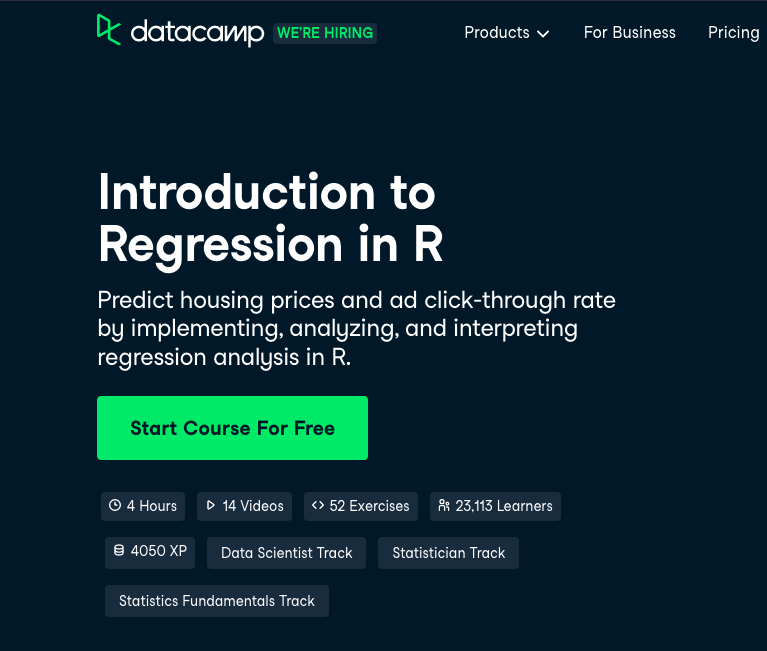In this course, you’ll learn to quantify model performance and diagnose problems with model fit to make predictions from your data.

Introduction to Regression in R

### Intermediate Regression in R

If you have sound R programming skills and expert knowledge of regression, then this course will bootstrap your abilities in using statistical models for Data Science.

This course is suitable for learners who have taken Introduction to Regression in R by DataCamp.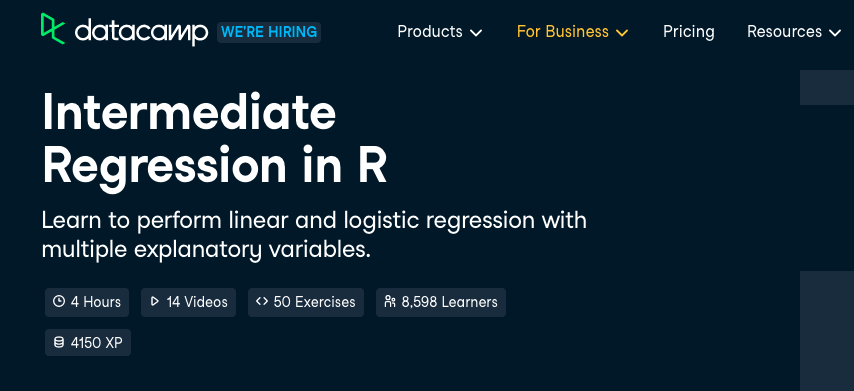By the end, you’ll have a deeper understanding of how linear and logistic regressions work by working on real-world datasets.

Intermediate Regression in R

### Correlation and Regression in R

This course is suitable for learners who have understanding and some experience in doing Exploratory Data Analysis in R Programming.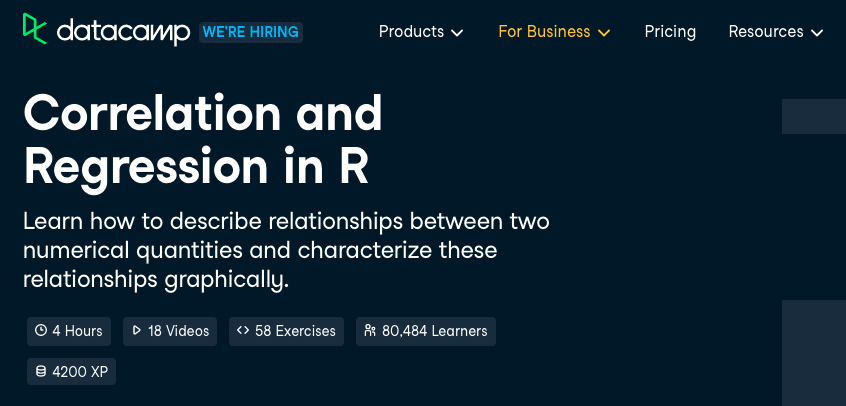By the end, you’ll not only have mastered the techniques for bivariate relationships but also gained advanced skills in correlation, Simple Linear Regression, interpreting regression models, Model Fit.

Correlation and Regression in R

### Introduction to Statistical Modeling in R

This intermediate-level course introduces learners to the most important methodologies in statistics by using R programming for building models.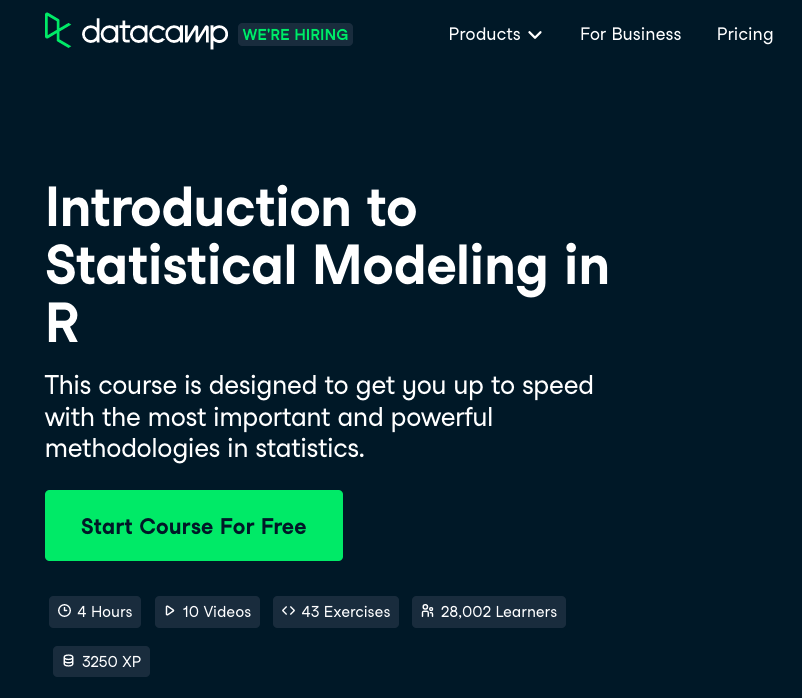By the end, you will have skills in designing, training, and Evaluating Models, plus assessing prediction performance, exploring data with models, covariates and effect size

Introduction to Statistical Modeling in R

### Intermediate Statistical Modeling in R

This course is suitable for learners with a basic knowledge of statistics and experience in R Programming.

You will learn about sampling variability and mathematical transforms, and the implications of collinearity.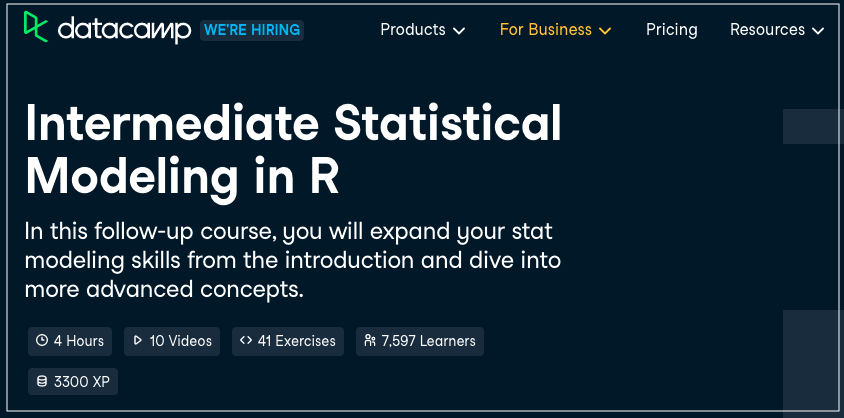Through the interactive exercises, you’ll dive deeper to a look at effect size and interaction, and then understand the concepts of a total and partial change.

Intermediate Statistical Modeling in R

### Statistical Modeling for Data Science Applications Specialization

This intermediate-level specialization teaches advanced statistical modelling techniques and equips learners with the functional knowledge for Data Science.

This specialization is suitable for learners who have a basic knowledge of R language, calculus, linear algebra, and probability theory.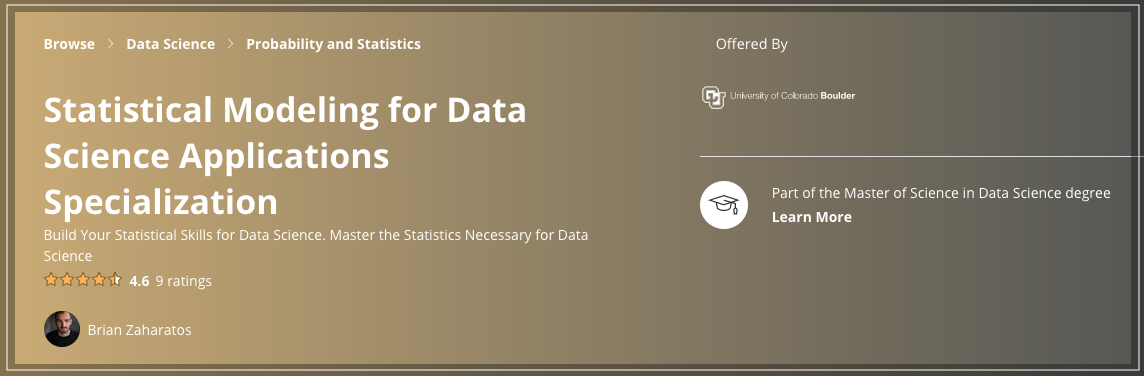By the end, you will have gained skills in Linear Model, R Programming, Statistical Model, Regression, Calculus, Probability, and Linear Algebra

Statistical Modeling for Data Science Applications Specialization

### Data Science: Statistics and Machine Learning Specialization

This high-rated specialization is highly recommended for learners to gain Statistical and Machine Learning skills for Data Science with R.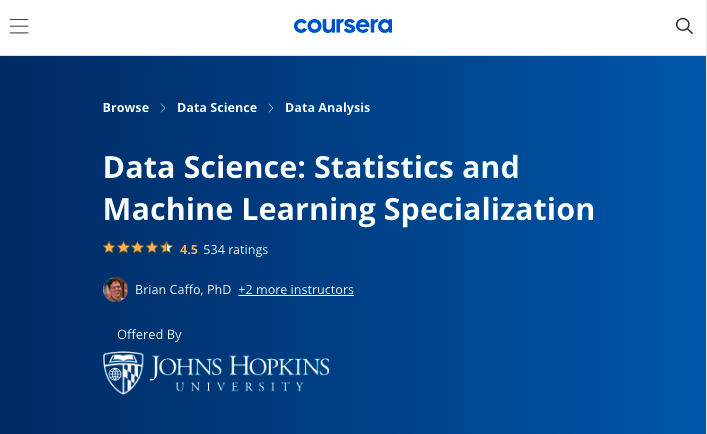Upon successful completion, you will have gained skills in Machine Learning, GitHub, R Programming, Regression Analysis, Data Visualization, Statistics, Statistical Inference, Statistical Hypothesis Testing, Model Selection, Generalized Linear Model, Linear Regression, and Random Forest.

Data Science: Statistics and Machine Learning Specialization

This course is suitable for learners who have intermediate R Programming skills and excellent knowledge of statistics.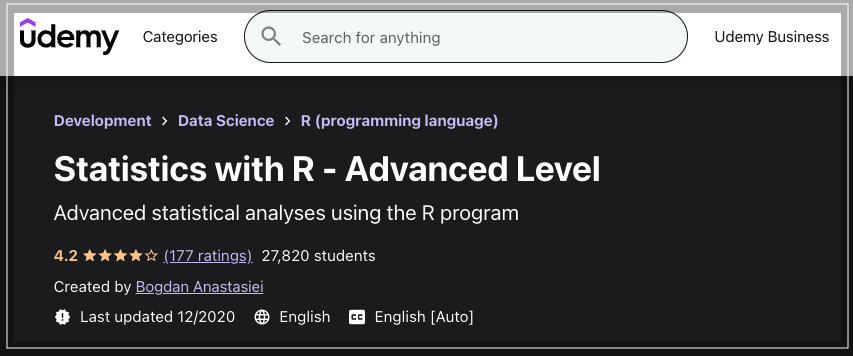Upon completion, you’ll be able to analyze covariance in the mixed analysis of variance, run the cluster analysis (k means and hierarchical), run the simple and multiple discriminant analysis, execute the binomial logistic regression, and more

### Advanced Linear Models for Data Science 1: Least Squares

In this course, learners will gain a firm foundation in a linear algebraic treatment of regression modelling.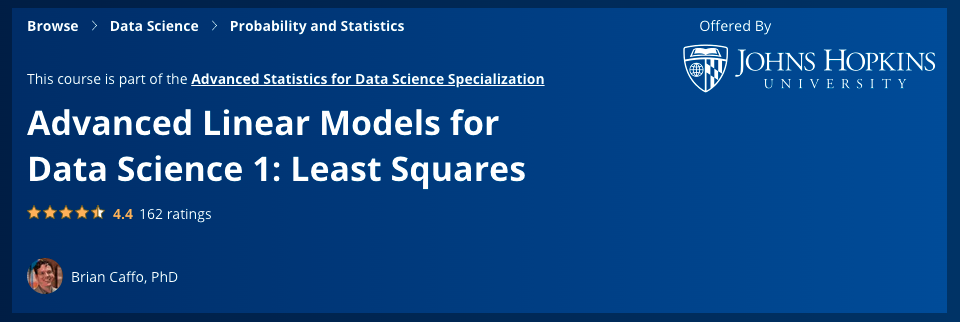Through the series of guided lectures, exercises, readings and discussions, you become equipped with the Sound Data Science skills in Linear Regression, R Programming, and Linear Algebra.

Advanced Linear Models for Data Science 1: Least Squares

### Advanced Linear Models for Data Science 2: Statistical Linear Models

In this course, you’ll dig much deeper to understand expected values, multivariate normal distribution, Distributional results, and Residuals.

This course is suitable for learners with a background in R and college-level statistics and Math.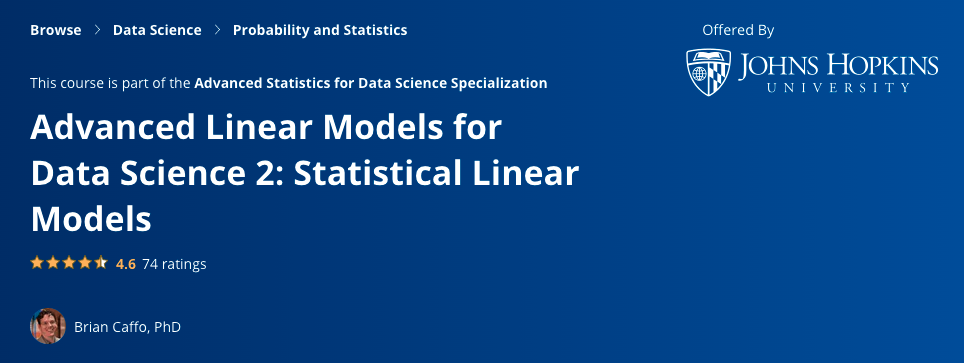You’ll go a little further in this course to gain a solid understanding of models for Data Science.

Advanced Linear Models for Data Science 2: Statistical Linear Models

### Advanced Statistics for Data Science Specialization

In this Advanced-level Specialization by Johns Hopkins University, you’ll become familiar with the fundamental concepts in probability and statistics, data analysis, matrix algebra, and linear models for Data Science.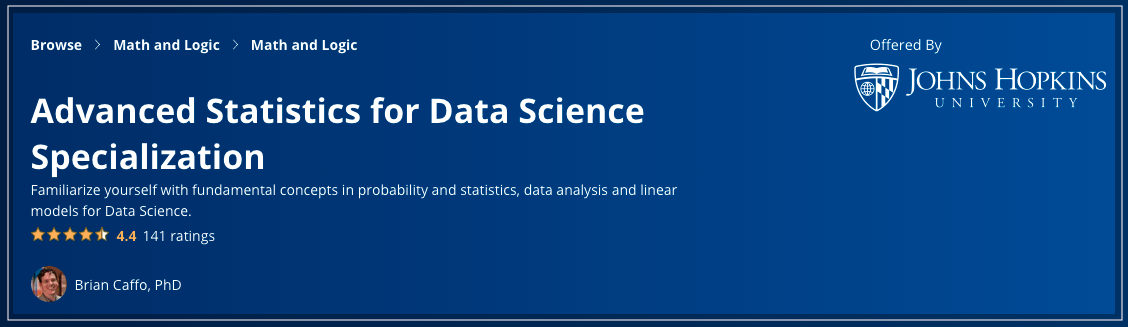Courses in this Specialization include:

• Mathematical Biostatistics Boot Camp
• Mathematical Biostatistics Boot Camp 2
• Advanced Linear Models for Data Science 1: Least Squares
• Advanced Linear Models for Data Science 2: Statistical Linear Models
Advanced Statistics for Data Science Specialization

### Practicing Statistics Interview Questions in R

In this course, you’ll prepare for the most frequently covered Statistics topics for Data Science to tackle problems in R Programming.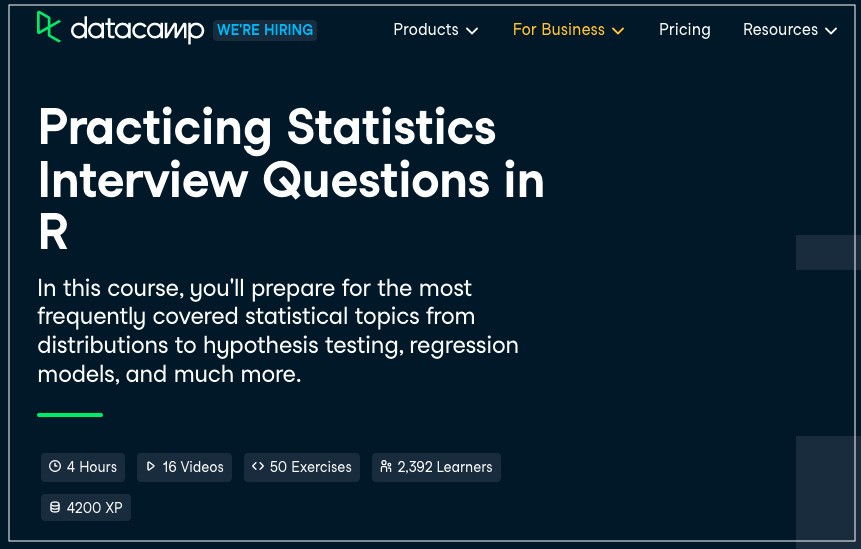By the end, you’ll have covered the key concepts and learned distributions to hypothesis testing, regression models, and more.

Practicing Statistics Interview Questions in R

Closing Note

Thanks for making it to the end...

If you're earnestly looking to get involved in Data Science, we highly suggest saving these recommended resources.

Contributors
Editors
No spam. In-depth analysis, expert opinions, startup perks, and resources to bootstrap your growth.
Oops! Something went wrong while submitting the form.

## Trending Stacks

Please bear with us, an update is coming soon.
Data Engineering
Master Data Engineering with these powerful tools, techniques, and resources.
Data Science
Discover a wealth of data science resources to elevate your analytical skills.
Cloud Computing
Elevate your cloud computing skills with up-to-date resources.